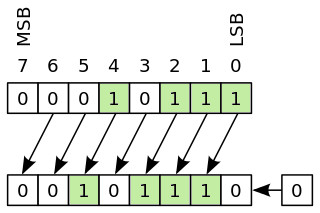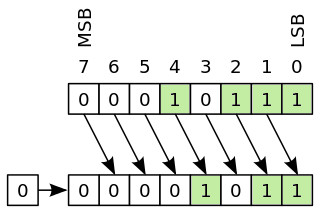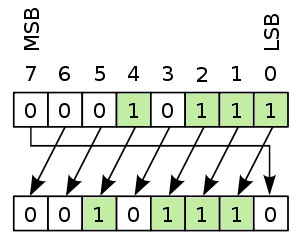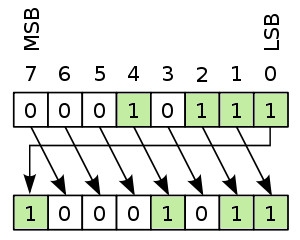# Bit shift and bit rotation algorithms with Scilab implementation

In Computer Engineering and in Systems with Microcontrollers areas of interest, performing bit operations is quite usual. In this tutorial we are going to learn what bit shifting and bit rotation means, an how to implement some simple algorithms in Scilab.

We are going to focus on:

• left / right bit shifting
• left / right bit rotation

For simplicity, in our examples, we are going to use only unsigned (positive) integers.

Any decimal number can be represented in binary format and vice-versa. To recall how to perform a decimal to binary and back to decimal conversion, read the articles Decimal to Binary Conversion and Binary to Decimal Conversion. Further, for a better understanding on the types of number representations, read the article Numbers Representation Systems – Decimal, Binary, Octal and Hexadecimal.

For an easy conversion from decimal to binary, we can use the Scilab functions dec2bin() and bin2dec(). For example, the decimal value 60 is represented in binary as 00111100:

--> dec2bin(60,8)

ans =
00111100

-->

--> bin2dec('00111100')

ans =
 60.

-->

The number 8 in the dec2bin() function states how many bits we use to represent the binary notation.

Bit shifting and bit rotation means moving bits from one position to another. There are similarities between bit shifting and bit rotation, the former having the property of keeping all the values of the bits, not loosing any.

### Left and right bit shifting

Bit shifting means moving the position of the bits in the left direction or in the right direction. For example, if we write in binary form the decimal number 23, we get 00010111. If we shift all bits left with one position, we’ll get 00101110. Bit 7 (MSB) was replaced by bit 6, bit 6 by bit 5 and so on.

Generally speaking, when shifting bits to left, bit n is replaced by bit n-1 and LSB becomes 0.(see image below).Image: Bit shift left (one position)

Since bit 0 was moved into position 1, a new bit 0 is stuffed in, with the value 0. After shifting, with one position, we got a new binary number. If we convert it to decimal, we get:

--> bin2dec('00101110')

ans =
 46.

-->

Our initial decimal number was 23, after shifting the bits left with one position we got 46, which is twice the initial value. Let see what happens if we shift the bits left but with two positions. We’ll get the binary number 01011100, which in decimal is:

--> bin2dec('01011100')

ans =
 92.

-->

Again, the final number 92 is twice the initial one (46). We can now conclude that left bit shifting with n positions means multiplying the initial number with 2n.

$N \cdot 2^n \leftarrow N$

What about right bit shifting? As the name suggests, the bits are moved to the right. Generally speaking, when shifting bits to the right, bit n is replaced by bit n+1 and MSB becomes 0 (see image below).Image: Bit shift right

If we take the same example, decimal 23 in binary is 00010111. Shifting to the right with one position will result in the binary number 00001011. Converting to decimal representation gives 11:

--> bin2dec('00001011')

ans =
 11.

-->

Let’s shift the bits right but with two positions. Our binary number is now 00000101 which in decimal notation is  5.

--> bin2dec('00000101')

ans =
 5.

-->

Right bit shifting is the equivalent of dividing the decimal number with 2n, where n is the number of positions to shift. Only the integer part of the division is kept, 23 shifted right with one position gave 11, which is the integer part of 23/2 = 11.5.

$N \rightarrow \text{integer part of }\frac{N}{2^n}$

### Scilab implementation of left and right bit shift

Scilab does not contain embedded functions for bit shifting. To overcome this limitation we are going to implement a custom Scilab function for bit shifting, defined as:

y=bitshift(str,dir,n,bit)

where:

str – string, defines the binary representation of a decimal number
dir – string, represents the direction of the bit shifting; can take only two values 'l' for left or 'r' for right
n – scalar, defines the number of positions to shift
bit – scalar, represents the number of bits for the output; can take only the following values: 8, 16, 32 or 64.
y – string, the return of the function, represents the binary number after the bit shift operation

Open a new Scilab script file and copy and paste the instructions below:

function y=bitshift(str,dir,n,bit)
dec=bin2dec(str);
switch bit
case 8
if dir=='r' then
y=dec2bin(uint8(dec/(2^n)),bit);
elseif dir=='l' then
y=dec2bin(uint8(dec*(2^n)),bit);
end
case 16
if dir=='r' then
y=dec2bin(uint16(dec/(2^n)),bit);
elseif dir=='l' then
y=dec2bin(uint16(dec*(2^n)),bit);
end
case 32
if dir=='r' then
y=dec2bin(uint32(dec/(2^n)),bit);
elseif dir=='l' then
y=dec2bin(uint32(dec*(2^n)),bit);
end
case 64
if dir=='r' then
y=dec2bin(uint64(dec/(2^n)),bit);
elseif dir=='l' then
y=dec2bin(uint64(dec*(2^n)),bit);
end
end
endfunction


The Scilab functions uint8(), uint16(), uint32() and uint64() are used to format the decimal value on the corresponding number of bits as the function argument bit. For a better understanding of the if-else and switch-case routines, read the articles Scilab programming – IF ELSE conditional statements and Scilab programming – SELECT CASE conditional statements.

Save the script file as bitshift.sci and execute it. Now you can use it in the Scilab console.

Let’s shift left, one position, the binary number 00111100:

--> bitshift('00111100','l',1,8)

ans =
 01111000

-->

If we want to display the same result on 32 bits, we need to change the bit argument as:

--> bitshift('00111100','l',1,32)

ans =
 00000000000000000000000001111000

-->

Same binary number shifted right, four positions and displayed on 16 bits:

--> bitshift('00111100','r',4,16)

ans =
 0000000000000011

-->

If we don’t know the binary representation of the number on which we want to bit shift, we can call the function replacing the str argument with the dec2str() function. Example:

--> bitshift(dec2bin(23),'l',2,8)

ans =
 01011100

-->

If we want to work only with decimal numbers, we can also put the function bitshift() as an argument for the bin2dec() function. Example:

--> bin2dec(bitshift(dec2bin(23),'l',2,8))

ans =
 92.

-->

### Left and right bit rotation

Bit rotation is similar to bit shift. It actually is bit shift but with a difference. The end bits, MSB or LSB are not any more lost and they come back on the other side of the string. Bit rotation is also known as circular bit shift.

When performing left bit rotation, with one position, bit n is replaced by bit n-1, and MSB comes at the back of the queue, replacing LSB.Image: Bit rotate left

Let’s take as example the decimal number 157. It’s binary representation is 10011101. If we rotate left, one position, we get 00111011, or decimal 59.

--> dec2bin(157)

 ans =
10011101

--> bin2dec('00111011')

 ans =
59.

-->

Right bit rotation has the same principle, the difference being that LSB is replacing MSB and bit n is replaced by bit n+1.Image: Bit rotate right

If we perform right bit rotation to the decimal number 59, we’ll get back our initial number 157. This is because we rotate back the bits on the initial positions, and 00111011 becomes 10011101.

### Scilab implementation of left and right bit rotation

Scilab doesn’t contain embedded functions for performing bit rotations. Thus, we are going to implement a custom Scilab function to perform bit rotation.

An efficient way of doing it is to split the bit rotation into 3 steps:

1. left bit shift by n positions
2. right shift by (NB – n) positions, where NB is the number of bits (8, 16, 32 or 64)
3. perform a logical OR between the results

For a better understanding of the algorithm, let’s assume that we have the following 8 bit binary number and we want to rotate it left with 3 positions.

b7 b6 b5 b4 b3 b2 b1 b0

1. shift left with 3 positions

b4 b3 b2 b1 b0 0 0 0

2. shift right (the initial representation) with 8-3=5 positions

0 0 0 0 0 b7 b6 b5

3. perform a bit OR operation

b4 b3 b2 b1 b0 b7 b6 b5

As you can see, bit rotation (also known as circular bit shift) can be performed using left, right bit shifts and bit OR operations. The same principle applies to right bit rotation but with reversed bit shift operations.

With this is mind, let’s design a Scilab function which performs bit rotations. The syntax of the function is:

y = bitrot(str,dir,n,bit)

where:

str – string, defines the binary representation of a decimal number
dir – string, represents the direction of the bit rotation; can take only two values, 'l' for left or 'r' for right
n – scalar, defines the number of positions to rotate
bit – scalar, represents the number of bits for the output; can take only the following values: 8, 16, 32 or 64.
y – string, the return of the function, represents the binary number after the bit shift operation

As you can see, the arguments of the bitrot() function are defined in the same way as the arguments of the bitshift() function.

Open a new Scilab script file and copy and paste the instructions below:

function y = bitrot(str,dir,n,bit)
if dir=='l' then
LS = bitshift(str,'l',n,bit);
RS = bitshift(str,'r',bit-n,bit);
elseif dir=='r' then
RS = bitshift(str,'r',n,bit);
LS = bitshift(str,'l',bit-n,bit);
end
y = dec2bin(bitor(bin2dec(LS),bin2dec(RS)),bit);
endfunction


For left and right bit shifting we are using the bitshift() function defined above. LS and RS are local variables representing the Left Side and Right Side of the bit OR operation.

To perform the bit OR operation we used the Scilab bitor() function. Notice that this function expects decimal inputs and returns also decimal outputs. That is why we used bin2dec() and dec2bin() functions for conversion.

Save the file as bitrot.sci and execute it. Now it can be called in the Scilab console.

Let’s test our function using the bit rotation example above:

--> bitrot('10011101','l',1,8)

 ans =
00111011

-->

--> bin2dec(ans)

 ans =
59.

-->

Let’s rotate the binary number 11111111 to the right, 4 positions and display the result on 32 bits:

--> bitrot('11111111','r',4,32)

 ans =
11110000000000000000000000001111

-->

If we want to use decimal numbers instead of binary representation strings, we can use the dec2bin() function instead of the str argument:

--> bitrot(dec2bin(65535),'l',22,32)

 ans =
11111111110000000000000000111111

-->

We can return the result of the bit rotation into a decimal value, by using the bitrot() function as argument for the bin2dec() function:

--> bin2dec(bitrot(dec2bin(127),'r',8,16))

 ans =
32512.

-->

Bit shift and bit rotate functions are used in cryptographic hash algorithms. For example SHA-256 (Security Hash Algorithm-256) contains several bit shift and rotate operations in its algorithm.

For any questions, observations and queries regarding this article, use the comment form below.

Don’t forget to Like, Share and Subscribe!

#### Ad Blocker Detected

Dear user, Our website provides free and high quality content by displaying ads to our visitors. Please support us by disabling your Ad blocker for our site. Thank you!